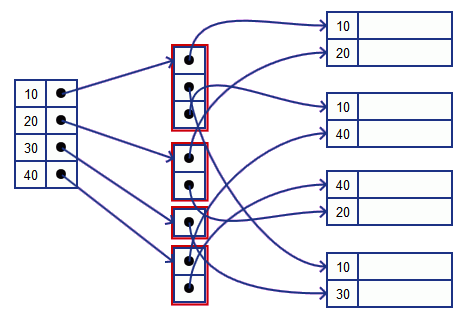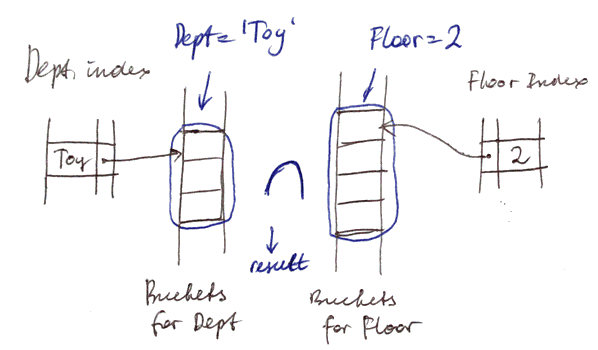# ML Wiki

## Buckets of Pointers

We add one more level of indirection for indexing

• we have index on buckets
• buckets are pointers to the actual tuples

So now we have

1. Dense Index where each value is stored once
2. Bucket list where we have multiple occurrences
• pointers to actual values
• should be sequential: i.e. ordered by the key
3. Actual blocks

Also saves space!

Example

•• index pointers point to the first occurrence of 10
• we read buckets down till there's no 10 anymore

Good for Range Queries

• These buckets are also useful for Range Queries

Suppose we have

• relation Emp(name, dept, floor)
• name is primary key (main index)
• dept and floor are secondary keys (Secondary Indexes on them)

Suppose we want to ask the following:

SELECT * FROM Emp where dept = 'Toy' AND floor = 2
•• need to compute the intersection of set of pointers (very fast)
• and this is before reading from disk